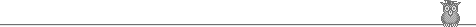Goto: Prof. King's page at Univ. of Florida.    Or: JK Homepage.
Modified: Friday, 12Nov2021     Printed: Friday, 21Jan2022
Page: http://squash.1gainesville.com/Include/thispage.shtml
JK focus Articles Fonts
testing Misc Navigation Schedule Teaching StanZas

Michael Dyck's Contradance Index LORs Pamphlets

PAST-COURSES Footnote, good books
SeLo 2022g LinA 2022t
SeLo 2021t Plex 2021t
SeLo 2021g DfyQ 2021g
SeLo 2020t, both sections Combinatorics 2017-2018
Algebra.1 2019t NT&Crypto 2019g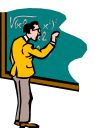Chronological list of courses taught 2003–ThePresent

…by Prof. JLF King at Univ. of FL. For just course titles, a complete 1990–ThePresent list is available.

Spring 2022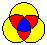Sets & Logic. Guides students on how to read/produce proofs, learning some of the language of modern Mathematics. My section will focus on problem-solving; both Putnam and USAMO problems will be used as examples. Both Induction and the Pigeon-hole Principle (a counting argument) will be introduced early in the semester.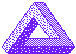(theoretical) Linear Algebra. Matrices, determinants, Gauss-Jordan algorithm, eigenvalues/vectors, matrix diagonalization, various matrix decompositions.

Autumn 2021Sets and Logic, 2021Autumn.
Guides students on how to read/produce proofs, learning some of the language of modern Mathematics. My section will focus on problem-solving; both Putnam and USAMO problems will be used as examples.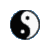Intro/Elem. Complex variables . How is complex differentiability connected to power-series expansion?

Spring 2021Sets and Logic. Guides students on how to read/produce proofs, learning some of the language of modern Mathematics. My section will focus on problem-solving; both Putnam and USAMO problems will be used as examples. Both Induction and the Pigeon-hole Principle (a counting argument) will be introduced early in the semester.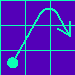Elem. Differential Eqns.

Linear, separable, autonomous DEs, intro to Differential Operators, for the bright, motivated, hard-working student. Time permitting, we'll introduce the Matrix Exponential as a way of solving interconnected linear DEs.

Prospective DiffyQ students should seriously review High-School mathematics (eqns of lines, parabolas; quadratic formula; sum of a geometric series) before or during Add/Drop.

Autumn 2020Sets and Logic, 2020Autumn.
Two sections, taught online due to COVID-19.
Guides students on how to read/produce proofs, learning some of the language of modern Mathematics. My section will focus on problem-solving; both Putnam and USAMO problems will be used as examples. Both Induction and the Pigeon-hole Principle (a counting argument) will be introduced early in the semester.

Spring 2020Sets and Logic, 2020g.Elem. Differential Eqns, 2020g

Linear, separable, autonomous DEs, intro to Differential Operators, for the bright, motivated, hard-working student. Time permitting, we'll introduce the Matrix Exponential as a way of solving interconnected linear DEs.

Prospective DiffyQ students should seriously review High-School mathematics (eqns of lines, parabolas; quadratic formula; sum of a geometric series) before or during Add/Drop.

Autumn 2019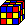Abstract Algebra 1 . An introduction to Groups, primarily, with some discussion of Rings and Fields. This is for the motivated hard-working ambitious student who likes structures, games, puzzles ...and Thinking in general.Sets and Logic, 2019t.

Spring 2019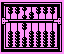Number Theory & Mathematical Cryptography .

This is a special topics course and is only offered once every 3 years or so. Also... NT&Math-Crypto counts as an Upper-division math-elective.

This course is an introduction to coding theory in general, and Mathematical Cryptography in particular. It does not assume a previous Number Theory course, only asking that the student do a bit of reading before the semester begins. (The webpage has a few suggestions.)Elem. Differential Eqns., 2019g.

Autumn 2018Sets and Logic . Helps students to read and produce proofs, and learn the basic language of modern Mathematics.Elem. Differential Eqns. Linear, separable, autonomous DEs, intro to Differential Operators, for the bright, motivated, hard-working student. Time permitting, we'll introduce the Matrix Exponential as a way of solving interconnected linear DEs.

Summer-B 2018Number Theory 1, of Summer-B 2018. Modular arithmetic, Chinese Remainder Thm, Legendre/ Jacobi symbols, Quadratic reciprocity, Fermat's SoTS thm, Lagrange 4-square thm, overview of Multiplicative Fncs.

Spring 2018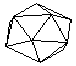Combinatorics II:

Continuing with Graph Theory, proceeding to Generating-Functions, followed by some more advanced material. Depending on student interest, we may cover some Combinatorial Game Theory.Elem. Differential Eqns. Linear, separable, autonomous DEs, differential operators, for the bright, motivated, hard-working student. Possibly, the Matrix Exponential method for solving interconnected linear DEs.

Autumn 2017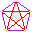Combinatorics I: Methods of counting, enumeration, bijective proofs.

The 1st semester (Autumn) will progress up to Graph Theory.

The 2nd semester (Spring 2018) will start with Generating-Functions, continue with Graph Theory, and some more advanced material. Depending on student interest, we may cover some Combinatorial Game Theory.Elem. Differential Eqns. Linear, separable, autonomous DEs, intro to differential operators.

Spring 2017Intro/Elem. Complex variables . How is complex differentiability connected to power-series expansion?Sets and Logic . Helps students to read and produce proofs, and learn the basic language of modern Mathematics.
[Sabbatical in Autumn 2016.]

Autumn 2016
On sabbatical in Autumn 2016.

Summer-B 2016Number Theory 1, of Summer-B 2016. Modular arithmetic, Chinese Remainder Thm, Legendre/ Jacobi symbols, Quadratic reciprocity, Fermat's SoTS thm, Lagrange 4-square thm, basic cryptography (Diffie-Hellman, RSA). Assumes no previous knowledge of Number Theory.

Spring 2016(theoretical) Linear Algebra. Matrices, determinants, Gauss-Jordan algorithm, eigenvalues/vectors, matrix diagonalization, various matrix decompositions.Number Theory & Mathematical Cryptography .

This is a special topics course and is only offered once every 3 years or so. Also... NT&Math-Crypto counts as an Upper-division math-elective.

This course is an introduction to coding theory in general, and Mathematical Cryptography in particular. It does not assume a previous Number Theory course, only asking that the student do a bit of reading before the semester begins. (The webpage has a few suggestions.)

Autumn 2015(theoretical) Linear Algebra. Matrices, determinants, Gauss-Jordan algorithm, eigenvalues/vectors, matrix diagonalization, various matrix decompositions.Elementary Differential Equations MAP2302  section 5609 : Beginning differential eqns for the bright, motivated, hard-working student.

Spring 2015Elementary Differential Equations, MAP2302  section 3145  (4th period): Beginning differential eqns for the bright, motivated, hard-working student.Sets and Logic: MHF3202  section 7860  (6th period, LIT201): Helps students to read and produce proofs, and learn the basic language of modern Mathematics.

Autumn 2014Elementary Differential Equations, MAP2302  section 4988  (4th period, LIT205): Beginning Differential Eqns for the bright, motivated, hard-working student.Elementary Differential Equations, MAP2302  section 5607  (5th period, LIT205): More Differential Eqns for the bright, motivated, even harder-working student!

Spring 2014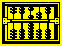Number Theory & Mathematical Cryptography, MAT4930 7554 (6th period, LIT221): This Special Topics course assumes basic NT (e.g, Euler-phi function, modular arithmetic, Fermat's Sum-of-Two-Square theorem).Sets and Logic, MHF3202  section 8768  (7th period, LIT203): Helps students to read and produce proofs, and learn the basic language of modern Mathematics.

Autumn 2013Elementary Differential Equations MAP2302  section 4689 : Beginning differential eqns for the bright, motivated, hard-working student.
There will be a test of prerequisite knowledge on Friday, 23Aug..Sets and Logic. Helps students to read and produce proofs, and learn the basic language of modern Mathematics. There will be a test of prerequisite knowledge on Friday, 23Aug..

Spring 2013Combinatorics II , will start with the clever notion of Generating Functions.Number Theory & Cryptography . This course is an introduction to coding theory in general, and Mathematical Cryptography in particular. It does not assume a previous Number Theory course, only asking that the student do a bit of reading before the semester begins. (The webpage has a few suggestions.)

Autumn 2012Combinatorics I , with many methods of counting. Note: I give test of prerequisite knowledge during Add/Drop. A practice exam is on our webpage.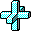Calculus III Careful treatment of multi-dimensional calculus. Note: I give test of prerequisite knowledge during Add/Drop. Our webpage has a practice exam.

Spring 2012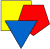Euclidean Geometry. A proof-based course covering a superset of: Theorems on Triangles (centroid, in-center, circum-center, ortho-center, Euler-line, Simson-Line), circles (Central-angle thm, Power-of-a-point), ruler/straightedge contructions and dissections of polygons. Matrix multiplication will be introduced for easy descriptions of transformations preserving Euclidean theorems. Time permitting, elem. Projective Geometry will be introduced, since many PG thms are also EG thms.Sets and Logic. Helps students to read and produce proofs, and learn the basic language of modern Mathematics. There was a test of prerequisite knowledge on Wedn, 11Jan.; our webpage has a practice exam.

Autumn 2011(theoretical) Linear Algebra. Matrices, determinants, Gauss-Jordan algorithm, eigenvalues/vectors, matrix diagonalization, various matrix decompositions.Sets and Logic . Helps students to read and produce proofs, and learn the basic language of modern Mathematics.

Spring 2011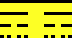Modern Analysis II . The continuation of a full-year course in Real Analysis. Time permitting, we will learn some Ergodic Theory (dynamical systems) this semester. Its undergrad number is MAA4227 6499 and grad is MAA5228 7059.Number Theory & Mathematical Cryptography: [MAT4930 7554] This Special Topics course assumes basic NT (e.g, Euler-phi function, modular arithmetic, Fermat's Sum-of-Two-Square theorem).

Autumn 2010Modern Analysis I
. A full-year course in Real Analysis. It uses the highly-regarded Baby Rudin text. Time permitting, I hope to discuss a bit of Ergodic Theory (dynamical systems) in the 2nd semester.(Abstract) Linear Algebra .

Spring 2010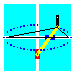Calculus II . Careful treatment of 1-dimensional calculus, with emphasis on Taylor's theorem and Taylor series.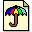Ergodic Theory and Dynamical Systems 2.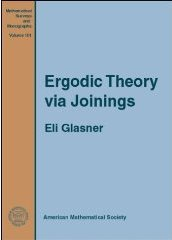Second semester of a full-year intro course in Dynamical systems, using the new-ish text by Glasner.

Autumn 2009Ergodic Theory and Dynamical Systems 1 . A full-year introductory course in Dynamical systems with emphasis on Ergodic theory (studies measure-preserving maps of a space to itself) and elementary Topological Dynamics (studies continuous maps of a compact metric-space to itself). As time permits, Symbolic dynamics may be studied.Sets and Logic . Helps students to read and produce proofs, and learn the basic language of modern Mathematics.

Autumn 2008 and Spring 2009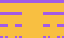Advanced Calculus for Engineers and (Physical) Scientists [ACES] Introductory Real Analysis on Euclidean Spaces. One might say that it is Calculus done right, on Rn, with rigorous definitions and proofs. (If time permits, we'll do an introduction to Metric Spaces, probably in the second semester.)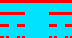Advanced Calculus (Theoretical) [ACT] Introductory Real Analysis on Metric Spaces. For students who plan to do graduate work in mathematics, this is the AdvCalc course to take.

Spring 2008Abstract Algebra 1 . An introduction to Groups, Rings and Fields. This is for the motivated hard-working ambitious student who likes games, puzzles ...and Thinking in general.Sets and Logic . Helps students to read and produce proofs, and learn the basic language of modern Mathematics.

Autumn 2007Number Theory & Elliptic Curve Cryptography is an undergraduate Special Topics course which is also appropriate for graduate students who have not had extensive Number Theory. This course does not require MAS4203 as prerequisite. All that is necessary is some preparatory reading from a free online NT text. What we cover will be partly determined by students' interests.

The central theme is Number Theory and codes of various kinds: Diffie-Hellman protocol, Huffman coding, Ziv-Lempel, Meshalkin isomorphism code, Elliptic Curve Codes. We will discuss various algorithms, such as repeated-squaring, and Shank's Baby-step Giant-step method for computing a Discrete logarithm.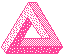Computational Linear Algebra . Matrices, determinants, Gauss-Jordan algorithm, eigenvalues/vectors, matrix diagonalization, various matrix decompositions.

My section of this course will have a test of prerequisite knowledge on Monday, 27Aug2007. The course webpage has a Sample Exam.

Spring 2007Number Theory 1, of Spring 2007. Modular arithmetic, Chinese Remainder Thm, Legendre/ Jacobi symbols, Quadratic reciprocity, Fermat's SoTS thm, Lagrange 4-square thm, basic cryptography (Diffie-Hellman, RSA). Assumes no previous knowledge of Number Theory.Computational Linear Algebra . Matrices, determinants, Gauss-Jordan algorithm, and various matrix decompositions.

Autumn 2006Number Theory 2 & Cryptography: A continuation of my NT1 with an emphasis on Mathematical Cryptography. Course NT1 is not a prerequisite; modular arithmetic and a little bit more is sufficient.Advanced Calculus This is an introductory Real Analysis course. One might say that it is "Calculus done right", with rigorous definitions and proofs. If time permits, we'll do an introduction to Metric Spaces.

Spring 2006Introduction to Number Theory, 1 , Spring 2006. This course is an introduction to elementary number theory. It assumes no previous knowledge of number theory.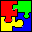Numbers & Polynomials . Textbook: Numbers & Polynomials by Prof. Kermit Sigmon. This course is run Moore Method, meaning that students prove all theorems, with enlightened guidance from the Professor.

Autumn 2005(theoretical) Linear Algebra . MAS4105.Abstract Algebra 1 An introduction to Groups, Rings and Fields.

Spring 2005
For the academic year Autumn 2004 through Spring 2005 I was on Research Leave.

Autumn 2004Prof. King will be on Research Leave for Autumn2004-Spring2005 and will not be teaching. (He does plan to be present!, working on a writing project.)

Spring 2004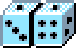Probability & Potential Theory 2 MAP6473 MWF 7 in FLO100. (Griffin-Floyd Hall.)Elementary Differential Equations MAP2302  section 3145 : Beginning differential eqns for the bright, motivated, hard-working student.

Autumn 2003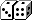Probability & Potential Theory 1 MAP6472 MWF2 in LIT217.Calculus III Careful treatment of multi-dimensional calculus.

Spring 2003Abstract Algebra 1 An introduction to Groups, Rings and Fields.Elementary Differential Equations Beginning differential eqns for the bright, motivated, hard-working student.

Autumn 2002Numbers&Polynomials Axioms of the real-number-system. Class is run Moore-method, with students collaborating to find the proofs.Honors Calculus III Careful treatment of multi-dimensional calculus.